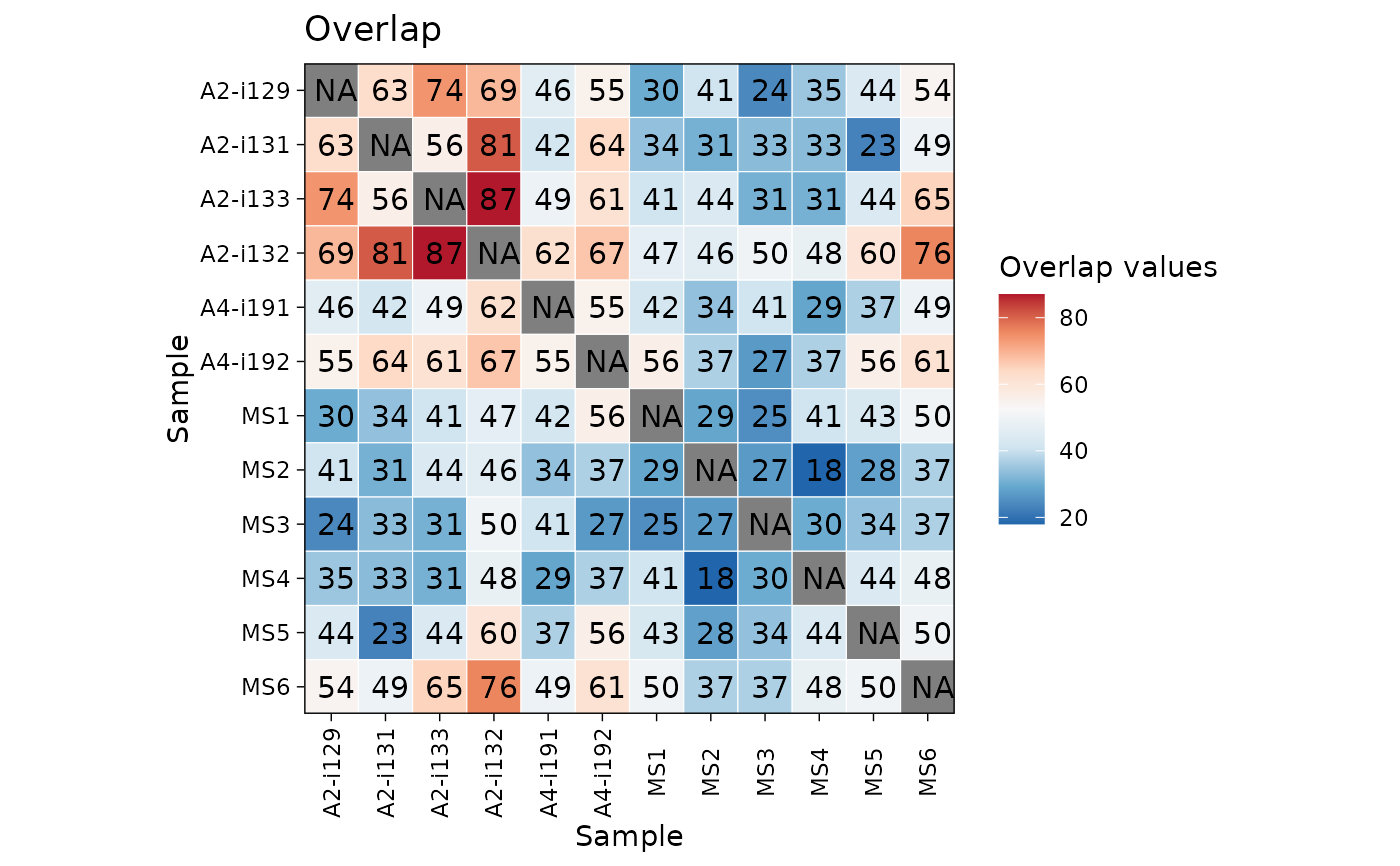Fast and easy visualisations of matrices or data frames with functions based on the ggplot2 package.

vis_heatmap(
.data,
.text = TRUE,
.scientific = FALSE,
.signif.digits = 2,
.text.size = 4,
.axis.text.size = NULL,
.labs = c("Sample", "Sample"),
.title = "Overlap",
.leg.title = "Overlap values",
.legend = TRUE,
.na.value = NA,
.transpose = FALSE,
...
)

## Arguments

.data

Input object: a matrix or a data frame.

If matrix: column names and row names (if presented) will be used as names for labs.

If data frame: the first column will be used for row names and removed from the data. Other columns will be used for values in the heatmap.

.text

If TRUE then plots values in the heatmap cells. If FALSE does not plot values, just plot coloured cells instead.

.scientific

If TRUE then uses the scientific notation for numbers (e.g., "2.0e+2").

.signif.digits

Number of significant digits to display on plot.

.text.size

Size of text in the cells of heatmap.

.axis.text.size

Size of text on the axis labels.

.labs

A character vector of length two with names for x-axis and y-axis, respectively.

.title

The The text for the plot's title.

.leg.title

The The text for the plots's legend. Provide NULL to remove the legend's title completely.

.legend

If TRUE then displays a legend, otherwise removes the legend from the plot.

.na.value

Replace NA values with this value. By default they remain NA.

.transpose

Logical. If TRUE then switch rows and columns.

...

Other passed arguments.

## Value

A ggplot2 object.

data(immdata)
ov <- repOverlap(immdata$data) vis_heatmap(ov)gu <- geneUsage(immdata$data, "hs.trbj")